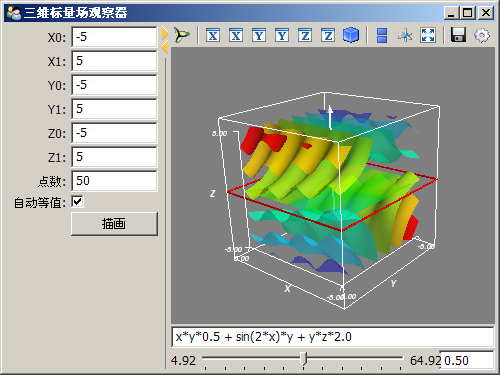# Mayavi-更方便的可视化

Mayavi2完全用Python编写，因此它不但是一个方便实用的可视化软件，而且可以方便地用Python编写扩展，嵌入到用户编写的Python程序中，或者直接使用其面向脚本的API：mlab快速绘制三维图。

## 用mlab快速绘图

```import numpy as np
from enthought.mayavi import mlab

x, y = np.ogrid[-2:2:20j, -2:2:20j]
z = x * np.exp( - x**2 - y**2)

pl = mlab.surf(x, y, z, warp_scale="auto")
mlab.axes(xlabel='x', ylabel='y', zlabel='z')
mlab.outline(pl)
```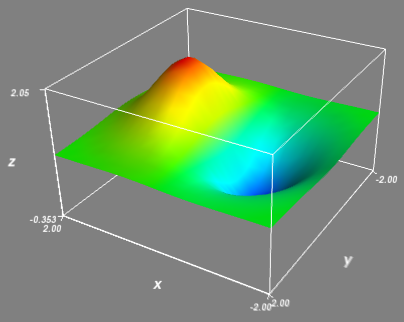```from enthought.mayavi import mlab
```

surf绘制的曲面在X-Y平面上的投影是一个等距离的网格，如果需要绘制更复杂的三维曲面的话，可以使用mesh函数。下面是mesh函数的一个例子：

```# -*- coding: utf-8 -*-
from numpy import *
from enthought.mayavi import mlab

# Create the data.
dphi, dtheta = pi/20.0, pi/20.0
[phi,theta] = mgrid[0:pi+dphi*1.5:dphi,0:2*pi+dtheta*1.5:dtheta]
m0 = 4; m1 = 3; m2 = 2; m3 = 3; m4 = 6; m5 = 2; m6 = 6; m7 = 4;
r = sin(m0*phi)**m1 + cos(m2*phi)**m3 + sin(m4*theta)**m5 + cos(m6*theta)**m7
x = r*sin(phi)*cos(theta)
y = r*cos(phi)
z = r*sin(phi)*sin(theta)

# View it.
s = mlab.mesh(x, y, z, representation="wireframe", line_width=1.0 )

mlab.show()
```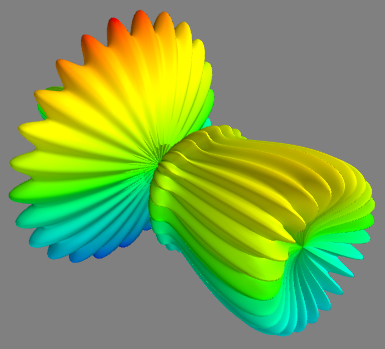mesh和surf类似，其三个数组参数x, y, z也是二维数组，他们相同下标的三个元素组成曲面上某点的三维坐标。点之间的连接关系(边和面)由其在x,y,z数组中间的位置关系决定。

• surface : 缺省值，绘制曲面

• wireframe : 绘制边线，将dphi, dtheta的改为较大值，例如pi/20之后，调用 ：

```s = mlab.mesh(x, y, z, representation="wireframe", line_width=1.0 )
```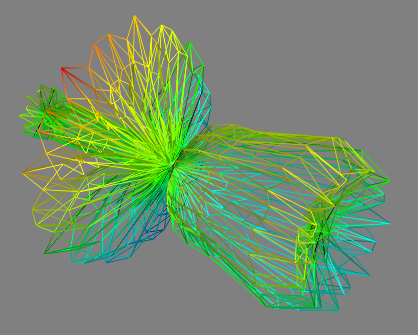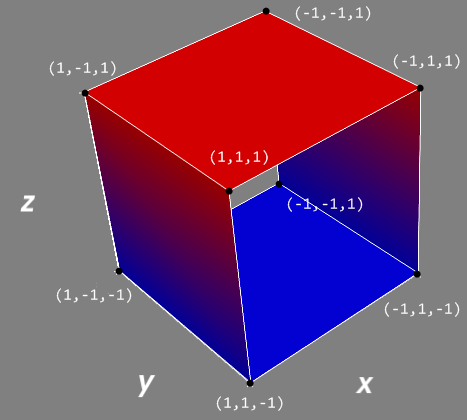x,y,z数组的定义如下：

```x = [[-1,1,1,-1,-1],
[-1,1,1,-1,-1]]

y = [[-1,-1,-1,-1,-1],
[1,1,1,1, 1]]

z = [[1,1,-1,-1,1],
[1,1,-1,-1,1]]
```

x, y, z数组对应坐标的元素组成三维坐标点，因此这三个数组实际描述的坐标点为：

```[
[(-1, -1, 1), (1, -1, 1), (1, -1, -1), (-1, -1, -1), (-1, -1, 1)]
[(-1, 1, 1), (1, 1, 1), (1, 1, -1), (-1, 1, -1), (-1, 1, 1)]
]
```

```(-1,-1,1),(1,-1,1),(-1,1,1),(1,1,1)
```

```(1,-1,1),(1,-1,-1),(1,1,1),(1,1,-1)
```

• points3d, plot3d : 给它们传递的3个坐标数组x,y,z都是一维的，因此这两个函数绘制出来的是三维空间中的一系列点(points3d)，或者是一条曲线(plot3d)。下图是采用plot3d绘制的洛仑兹吸引子的轨迹：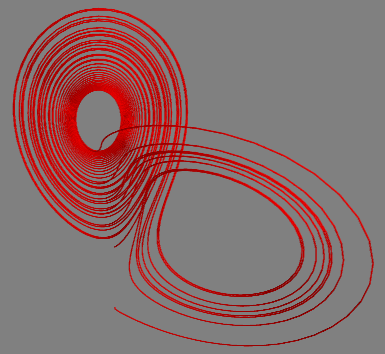plot3d函数绘制的洛仑兹吸引子，曲线使用很细的圆管绘制

```mlab.plot3d(track1[:,0], track1[:,1], track1[:,2],color=(1,0,0), tube_radius=0.1)
```

• imshow, surf, contour_surf : 这三个函数都可以接收一个二维数组s，以其第一轴的下标为X轴坐标，第二轴的下标为Y轴坐标。imshow函数将此二维数组当作一个图片显示，每点的颜色为数组s的每个元素的值。surf函数则将此二维数组绘制成三维空间中的曲面，数组中每个元素的值为点的Z轴坐标。contour_surf则绘制二维数组的等高线。下面是imshow函数的绘制结果(所使用的数组和前面surf函数的例子相同)：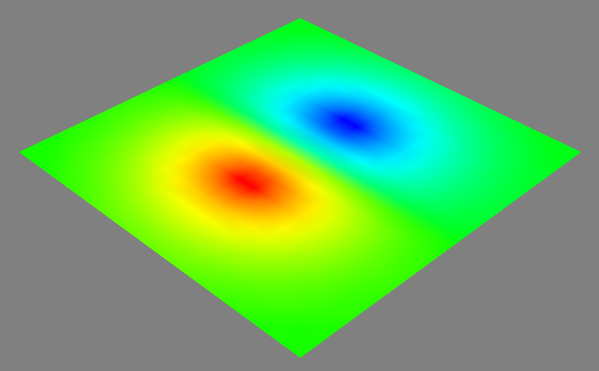imshow函数将二维数组绘制成图像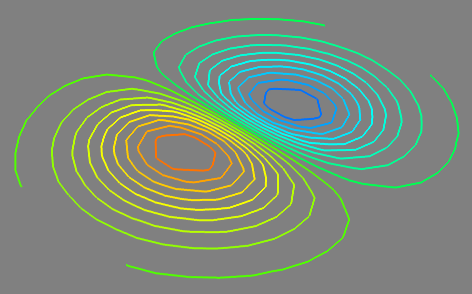contour_surf函数绘制二维图像的等高线

## 将Mayavi嵌入到界面中

Mayavi除了能够单独作为应用程序使用之外，也可以通过traits属性嵌入到TraitsUI制作的用户应用程序的界面中去，下面的程序演示了这一过程：

```# -*- coding: utf-8 -*-
from enthought.traits.api import *
from enthought.traits.ui.api import *
from enthought.tvtk.pyface.scene_editor import SceneEditor
from enthought.mayavi.tools.mlab_scene_model import MlabSceneModel
from enthought.mayavi.core.ui.mayavi_scene import MayaviScene

class DemoApp(HasTraits):
plotbutton = Button(u"绘图")
scene = Instance(MlabSceneModel, ()) # mayavi场景

view = View(
VGroup(
Item(name='scene',
editor=SceneEditor(scene_class=MayaviScene), # 设置mayavi的编辑器
resizable=True,
height=250,
width=400
),
'plotbutton',
show_labels=False
),
title=u"在TraitsUI中嵌入Mayavi"
)

def _plotbutton_fired(self):
self.plot()

def plot(self):
g = self.scene.mlab.test_mesh()

app = DemoApp()
app.configure_traits()
```

MlabSceneModel类是包装整个mlab的场景的模型，它是属于模型(Model)方面的东西，因此程序中通过:

```scene = Instance(MlabSceneModel, ())
```

```Item(name='scene',
editor=SceneEditor(scene_class=MayaviScene), # 设置mayavi的编辑器
resizable=True,
height=250,
width=400
)
```

SceneEditor是用来创建场景编辑器的工厂类，通过关键字scene_class指定真正创建场景对象类MayaviScene。

```g = self.scene.mlab.test_mesh()
```

scene.mlab和前面所介绍的mlab库一样使用，我们调用其test_mesh测试函数，快速在scene中创建一个如下图所示的很酷的曲面体。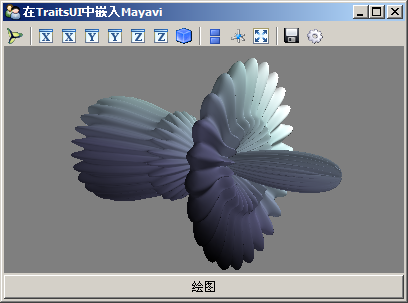```# 产生三维网格
x, y, z = mgrid[
self.x0:self.x1:1j*self.points,
self.y0:self.y1:1j*self.points,
self.z0:self.z1:1j*self.points]
scalars = eval(self.function) # 根据函数计算标量场的值
```

```self.scene.mlab.clf() # 清空当前场景
```

```# 绘制等值面
g = self.scene.mlab.contour3d(x, y, z, scalars, contours=8, transparent=True)
g.contour.auto_contours = self.autocontour
self.scene.mlab.axes() # 添加坐标轴

# 添加一个X-Y的切面
s = self.scene.mlab.pipeline.scalar_cut_plane(g)
cutpoint = (self.x0+self.x1)/2, (self.y0+self.y1)/2, (self.z0+self.z1)/2
s.implicit_plane.normal = (0,0,1) # x cut
s.implicit_plane.origin = cutpoint
```

```self.g = g
self.scalars = scalars
# 计算标量场的值的范围
self.v0 = np.min(scalars)
self.v1 = np.max(scalars)
```

```self.g.contour.auto_contours = self.autocontour
```

```if not self.g.contour.auto_contours:
self.g.contour.contours = [self.contour]
```